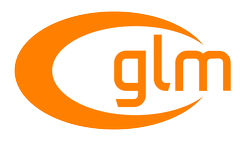0.9.9 API documentation
GLM_GTX_rotate_normalized_axis

Include <glm/gtx/rotate_normalized_axis.hpp> to use the features of this extension. More...

Functions

template<typename T , qualifier Q>
GLM_FUNC_DECL mat< 4, 4, T, Q > rotateNormalizedAxis (mat< 4, 4, T, Q > const &m, T const &angle, vec< 3, T, Q > const &axis)
Builds a rotation 4 * 4 matrix created from a normalized axis and an angle. More...

template<typename T , qualifier Q>
GLM_FUNC_DECL qua< T, Q > rotateNormalizedAxis (qua< T, Q > const &q, T const &angle, vec< 3, T, Q > const &axis)
Rotates a quaternion from a vector of 3 components normalized axis and an angle. More...

Detailed Description

Include <glm/gtx/rotate_normalized_axis.hpp> to use the features of this extension.

Quaternions and matrices rotations around normalized axis.

Function Documentation

 GLM_FUNC_DECL mat<4, 4, T, Q> glm::rotateNormalizedAxis ( mat< 4, 4, T, Q > const & m, T const & angle, vec< 3, T, Q > const & axis )

Builds a rotation 4 * 4 matrix created from a normalized axis and an angle.

Parameters
 m Input matrix multiplied by this rotation matrix. angle Rotation angle expressed in radians. axis Rotation axis, must be normalized.
Template Parameters
 T Value type used to build the matrix. Currently supported: half (not recommended), float or double.